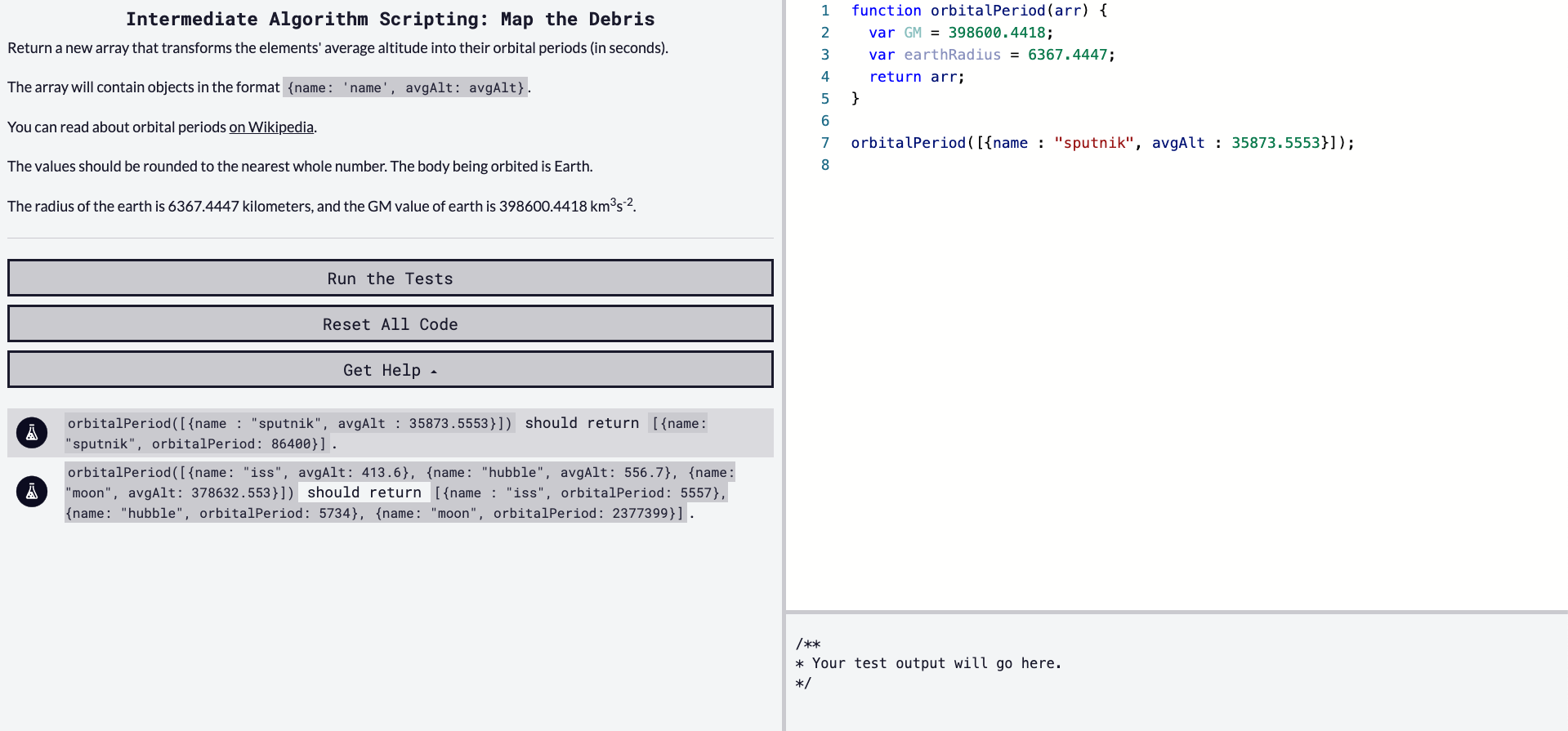## DEV Community

Viren B

Posted on • Originally published at virenb.cc

# Solving "Map the Debris" / freeCodeCamp Algorithm Challenges

** Post can also be found on virenb.cc **Let's solve freeCodeCamp's intermediate algorithm scripting challenge, 'Map the Debris'.

### Starter Code

``````function orbitalPeriod(arr) {
var GM = 398600.4418;
return arr;
}

orbitalPeriod([{name : "sputnik", avgAlt : 35873.5553}]);
``````

### Instructions

Return a new array that transforms the elements' average altitude into their orbital periods (in seconds).

The array will contain objects in the format `{name: 'name', avgAlt: avgAlt}`.

The values should be rounded to the nearest whole number. The body being orbited is Earth.

The radius of the earth is 6367.4447 kilometers, and the GM value of earth is 398600.4418 km3s-2.

### Test Cases

• `orbitalPeriod([{name : "sputnik", avgAlt : 35873.5553}])` should return `[{name: "sputnik", orbitalPeriod: 86400}]`.
• `orbitalPeriod([{name: "iss", avgAlt: 413.6}, {name: "hubble", avgAlt: 556.7}, {name: "moon", avgAlt: 378632.553}])` should return `[{name : "iss", orbitalPeriod: 5557}, {name: "hubble", orbitalPeriod: 5734}, {name: "moon", orbitalPeriod: 2377399}]`.

# Our Approach

The instructions for this challenge are short and to the point.

• Our input is an array of one or more objects.

• We have to return the same array of objects, but altering one of the key-value pairs (`avgAlt` -> `orbitalPeriod`).

• Make sure orbitalPeriod is calculated in seconds and rounded up to the nearest whole number.

The last challenge seems a bit hard. This formula is a little complex. But basically we have to do some math to calculate it, deleted `avgAlt`, and add in `orbitalPeriod` into each object in the array.

After a lot of Internet searching (formerly known as Googling - I don't like to feed into the search engine bias), I came across the correct formula, `T = 2 * pi * sqrt(r^3/GM); r = earthRadius + avgAlt`.

GM is provided in the instructions, as is the radius of the Earth. We can start by setting those variables.

``````const GM = 398600.4418;
``````

Now that we have our values and a formula, we can plug in those values and do some math. Once we have the orbital period value, we can add it to each object, and remove the `avgAlt`. We can loop over each object in the array using `map()`.

``````arr.map(obj => {
let oP = Math.round(2 * Math.PI * Math.sqrt(Math.pow(earthRadius + obj.avgAlt, 3) / GM));
// More stuff
})
``````

So we set a variable with the above formula. We use `Math.round()` as the instructions wanted us to round to the nearest whole number. Next we can delete `avgAlt` as we have used it in the formula. Then we can add `oP` to the object.

``````arr.map(obj => {
let oP = Math.round(2 * Math.PI * Math.sqrt(Math.pow(earthRadius + obj.avgAlt, 3) / GM));
// Delete avgAlt
delete obj.avgAlt;
obj.orbitalPeriod = op;
})
``````

We have updated the object(s) in `arr`. Lastly, we want to make sure to return `arr`.

# Our Solution

``````function orbitalPeriod(arr) {
var GM = 398600.4418;
arr.map(obj => {
let oP = Math.round(2 * Math.PI * Math.sqrt(Math.pow(earthRadius + obj.avgAlt, 3) / GM));
delete obj.avgAlt;
obj.orbitalPeriod = oP;
})
return arr;
}
``````

'Map the Debris' Challenge on fCC

freeCodeCamp

Donate to FCC!

Solution on my GitHub

DEV Community

## The JavaScript Brief

### 1. Top 5 MERN STACK projects to improve your practical understanding

Boost your MERN Stack development skills by undertaking interesting beginner projects. These five engaging projects cover web applications and range from social media website applications to geo-social networking maps. Hone your understanding and apply modern techniques backed up by hands-on experience.

### 2. How To Optimize Your React App’s Performance

Learn the best optimizing techniques to make your React applications faster and more efficient. Focusing on the identification of performance bottlenecks and common pitfalls to avoid, these optimization strategies will keep your applications running smoothly even when faced with growing complexity.

### 3. A story of let, const, object mutation, and a bug in my code

In the pursuit of bug-free code, explore an incident involving a mix-up between `const` and `let`, making sure your custom code works effectively with third

party documentation. Discover best practices on program flow and learn about JavaScript's unpredictable aspects to ensure your core code is robust.